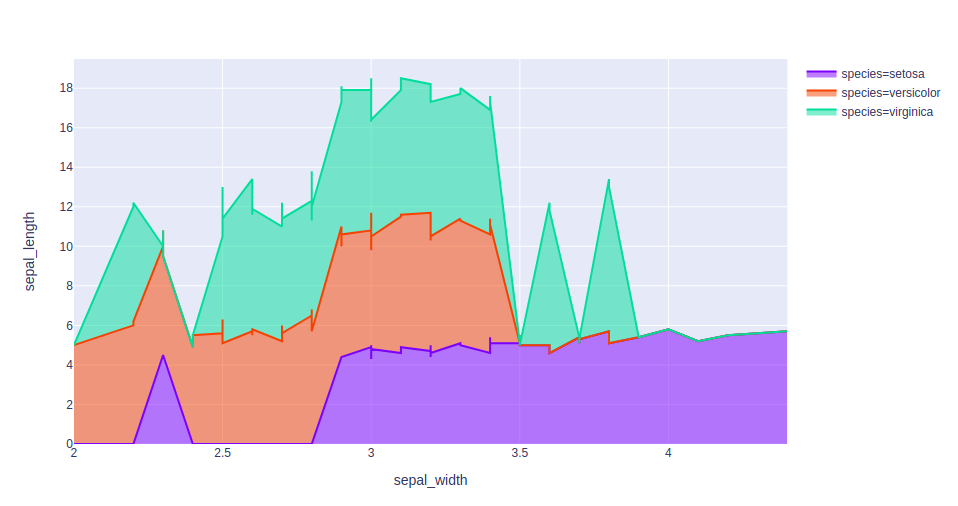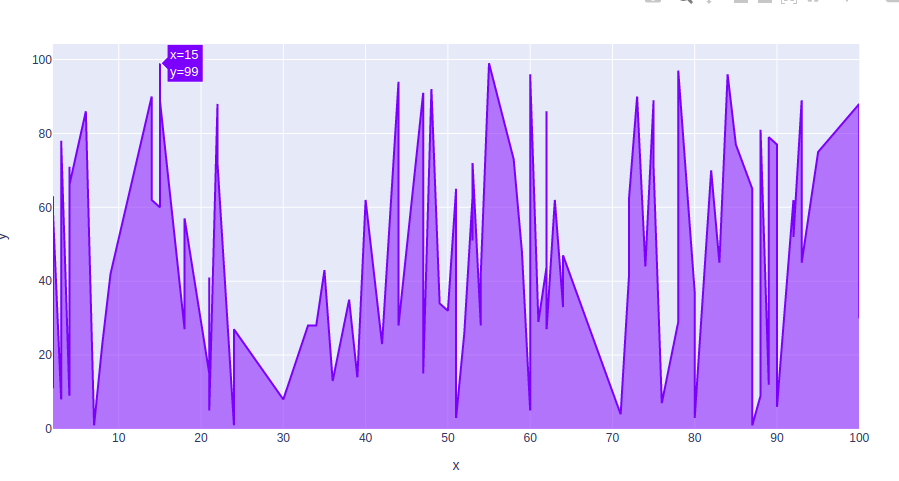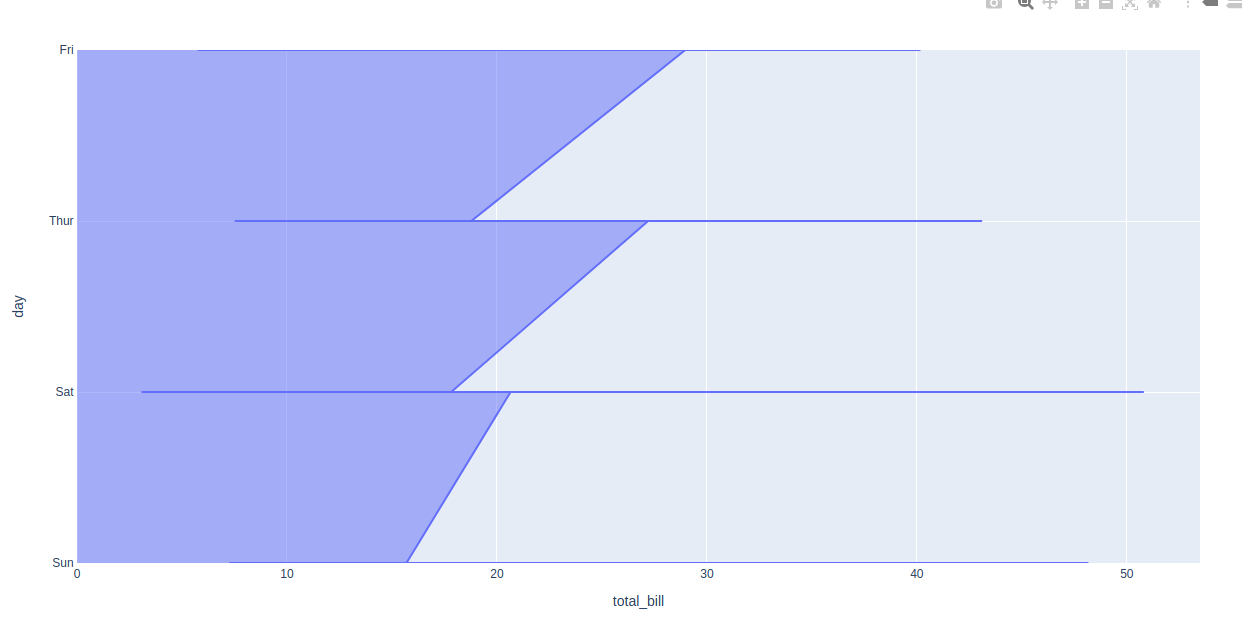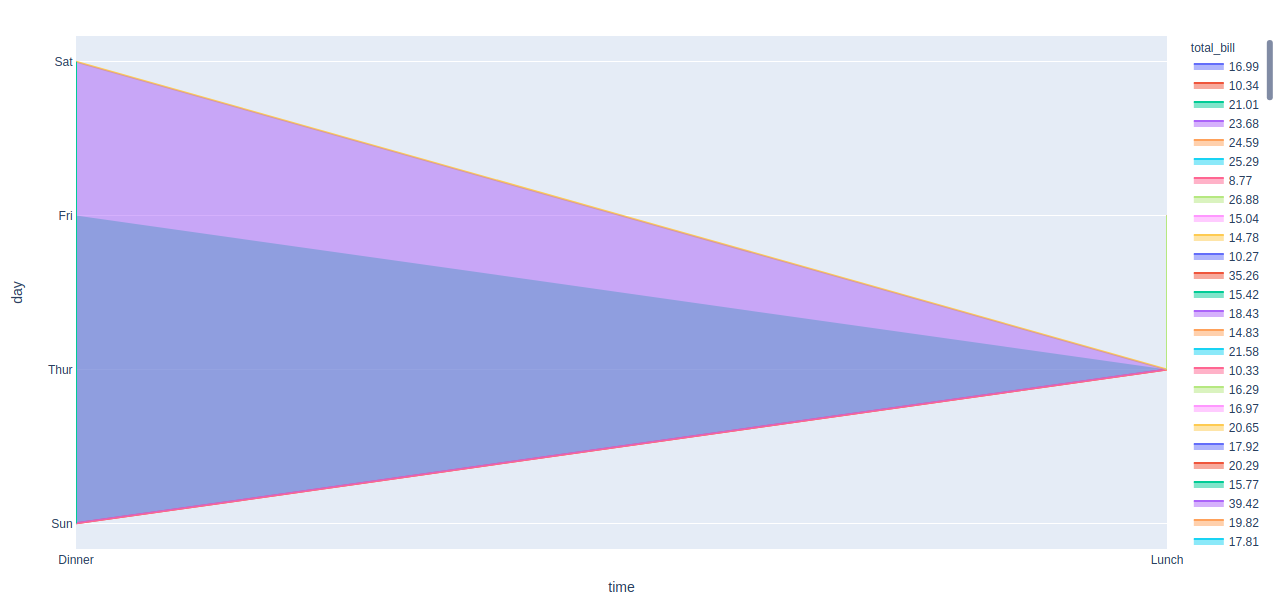Open In App

# Filled area chart using plotly in Python

Plotly is a Python library that is used to design graphs, especially interactive graphs. It can plot various graphs and charts like histogram, barplot, boxplot, spreadplot, and many more. It is mainly used in data analysis as well as financial analysis. plotly is an interactive visualization library.

## Filled Area Chart

Filled area plot is the easy-to-use, high-level articulation to plotly which completes a variety of types of data and produces easy-to-style figures. Each filled area harmonizes with one value of the column given by the line_group parameter. Filled area charts are most commonly used to show trends, rather than convey specific values. Two popular variations of Area Graphs are: grouped and Stacked Area Graphs.

Syntax: plotly.express.area(data_frame=None, x=None, y=None, line_group=None, color=None, hover_name=None, hover_data=None, custom_data=None, text=None, facet_row=None, facet_col=None, facet_col_wrap=0, animation_frame=None, animation_group=None, category_orders={}, labels={}, color_discrete_sequence=None, color_discrete_map={}, orientation=None, groupnorm=None, log_x=False, log_y=False, range_x=None, range_y=None, line_shape=None, title=None, template=None, width=None, height=None)

Parameters:

data_frame:  This argument needs to be passed for column names (and not keyword names) to be used. Array-like and dict are transformed internally to a pandas DataFrame.

x, y: Either a name of a column in data_frame, or a pandas Series or array_like object. Values from this column or array_like are used to position marks along the x and y axis in cartesian coordinates.

color: Either a name of a column in data_frame, or a pandas Series or array_like object. Values from this column or array_like are used to assign color to marks.

Example 1:

## Python3

 `import` `plotly.express as px` `df ``=` `px.data.iris()` `fig ``=` `px.area(df, x``=``"sepal_width"``, y``=``"sepal_length"``,``            ``color``=``"species"``,``            ``hover_data``=``[``'petal_width'``],)` `fig.show()`

Output:Example 2:

## Python3

 `import` `plotly.express as px``import` `numpy as np` `# creating random data through randomint``# function of numpy.random``np.random.seed(``42``)``  ` `random_x``=` `np.random.randint(``1``,``101``,``100``)``random_y``=` `np.random.randint(``1``,``101``,``100``)` `fig ``=` `px.area(x ``=` `random_x, y ``=` `random_y)``fig.show()`

Output:Example 3:

## Python3

 `import` `plotly.express as px`  `df ``=` `px.data.tips()``  ` `fig ``=` `px.area(df, x ``=``'total_bill'``, y ``=` `'day'``)``fig.show()`

Output:Example 4:

## Python3

 `import` `plotly.express as px`  `df ``=` `px.data.tips()``  `  `fig ``=` `px.area(df, x ``=``'time'``, y ``=` `'day'``,``              ``color``=``"total_bill"``)``fig.show()`

Output: